[BACK]
 Computers, Materials & ContinuaDOI:10.32604/cmc.2022.016488Article

Multi-Objective Grey Wolf Optimization Algorithm for Solving Real-World BLDC Motor Design Problem

1Department of Electrical and Electronics Engineering, GMR Institute of Technology, Rajam, 532127, Andhra Pradesh, India
2Rajasthan Rajya Vidyut Prasaran Nigam, Sikar, 332025, Rajasthan, India
3Department of Computer Science and Engineering, GMR Institute of Technology, Rajam, 532127, Andhra Pradesh, India
4German Jordanian University, Amman, 11180, Jordan
5Department of Mathematics, College of Arts and Sciences, Prince Sattam Bin Abdulaziz University, Wadi Aldawaser, 11991, Saudi Arabia
*Corresponding Author: Kottakkaran Sooppy Nisar. Email: n.sooppy@psau.edu.sa
Received: 03 January 2021; Accepted: 17 April 2021

Abstract: The first step in the design phase of the Brushless Direct Current (BLDC) motor is the formulation of the mathematical framework and is often used due to its analytical structure. Therefore, the BLDC motor design problem is considered to be an optimization problem. In this paper, the analytical model of the BLDC motor is presented, and it is considered to be a basis for emphasizing the optimization methods. The analytical model used for the experimentation has 78 non-linear equations, two objective functions, five design variables, and six non-linear constraints, so the BLDC motor design problem is considered as highly non-linear in electromagnetic optimization. Multi-objective optimization becomes the forefront of the current research to obtain the global best solution using metaheuristic techniques. The bio-inspired multi-objective grey wolf optimizer (MOGWO) is presented in this paper, and it is formulated based on Pareto optimality, dominance, and archiving external. The performance of the MOGWO is verified on standard multi-objective unconstraint benchmark functions and applied to the BLDC motor design problem. The results proved that the proposed MOGWO algorithm could handle nonlinear constraints in electromagnetic optimization problems. The performance comparison in terms of Generational Distance, inversion GD, Hypervolume-matrix, scattered-matrix, and coverage metrics proves that the MOGWO algorithm can provide the best solution compared to other selected algorithms. The source code of this paper is backed up with extra online support at https://premkumarmanoharan.wixsite.com/mysite and https://www.mathworks.com/matlabcentral/fileexchange/75259-multi- https://objective-non-sorted-grey-wolf-mogwo-nsgwo.

Keywords: BLDC motor; electromagnetics; metaheuristic; multi-objective grey wolf optimizer

1  Introduction

The BLDC motor is famous and preferable for real-world applications due to its electronic commutation feature. The BLDC motor is designed with a specific purpose, and it can be suitable for electronic or power device applications. The BLDC motor has high-quality permanent magnets in its fixed armature and eliminates problems associated with supplying current to the rotating armature. The electronic controller replaces the commutator/brush assembly in conventional DC motors, and its function is similar to power distribution by solid-state circuits . The BLDC motors offer various benefits over brushed motors, such as high torque per watt, high torque per weight ratio, low noise, high reliability, etc. The hall-effect position sensors or rotary encoders are used to locate the position of the rotor pole. The drawback associated with the BLDC motor is, high cost compared to the brushed DC motor, but this can be overcome by relatively high efficiency over the motor lifespan. The BLDC motor should be appropriately designed for real-time applications to achieve high motor efficiency. For the same, the optimal design parameters of the motor are required. Nevertheless, the performance characteristic of the motors can also be enhanced further by optimizing the design. Therefore, the optimization algorithm is required to obtain the optimal design parameters. It results in a reduction of the design period and overall cost. The BLDC motor design  is considered an optimization problem from the detailed literature, and the benchmark model for the same is formulated and presented in [3,4].

Finite element analysis (FEA) is not the only method available for the design of electromagnetic devices. Currently, the researchers are working on various global optimization techniques for the same. During the design process, analytical models are used, in which decisions are made with respect to the structure of the machine and materials. Certainly, analytical models have certain benefits over FEA: they are quicker and flexible with a large parameter and consider physical phenomena. The analytical models are also used to solve the design problems by solving the conflicting objective, i.e., determining the components' geometries from the device specifications. On the other hand, FEA can calculate the design requirements and allow iterative techniques to solve the problem [5,6] when geometric dimensions are known. However, the results produced by analytical models are less accurate, and critical skills are required to solve the problems. Therefore, metaheuristic methods are utilized to solve electromagnetic optimization problems. Nowadays, the field of global metaheuristics optimization is very active, and researchers are reporting numerous stochastic techniques for optimizing the constrained optimization problem in the continuous domain. Most of the metaheuristic techniques are inspired by nature. In the global optimization problem, the solutions may have multiple local optima, and it became a major challenge for the optimization algorithms. Therefore, many improvements are carried out in bio-inspired metaheuristics relating to evolutionary techniques [7,8] and swarm intelligence techniques  over the past few decades. Recently, global optimization trends with different metaheuristic techniques are active among researchers, including electromagnetic optimization problems . The BLDC benchmark problem has been solved with the nature-inspired Bat Algorithm (BA) , where multi-objective results are compared with Multi-Objective Krill Herd (MOKH)  and Non-Dominated Sorting Genetic Algorithm-Version II (NSGA-II) algorithm, including single-objective as well as {multi-objective} for BLDC wheel motor problem. The same problem has also been optimized through a Multi-Objective Particle Swarm Optimization (MOPSO) algorithm, and it is reported in . The NSGA-II is a high-speed and powerful technique for solving various multi-objective problems. The authors of  reported the Tabu search technique for solving the electromagnetic optimization problem in the continuous domain. However, the search technique stuck at local optima, and its convergence speed is very low. The authors of  reported a Genetic Algorithm (GA) to handle the BLDC motor design problem. The authors have also introduced the equivalent magnetic circuit concept along with GA for solving the same problem. However, the solution coverage is poor in the entire solution space. The authors of [18,19] optimized the core shape of the machine by introducing GA to reduce the cogging torque. However, the solution accuracy and the convergence speed are poor.

Various metaheuristic algorithms, such as Multi-objective Grey Wolf Algorithm (MOGWO) {[20,21]}, NSGA-II, Pareto Archived Evolution Strategy (PAES) , Multi-objective Firefly Algorithm (MOFA) , Multi-objective Bat Algorithm (MOBA) , Multi-objective Flower Pollination (MOFPA) , Multi-objective Shuffle Frog Leaping Algorithm (MOSFLA) , Multi-objective Particle Swarm Optimizer (MOPSO), Multi-objective Ant Lion Algorithm (MOALO) , Multi-objective Grasshopper Algorithm (MOGOA) , Multi-objective Salp Swarm Algorithm (MOSSA) , multi-objective heat transfer search algorithm , multi-objective modified adaptive symbiotic organisms search , hybrid heat transfer search and passing vehicle search optimizer , multi-objective modified heat transfer search , multi-objective passing vehicle search algorithm , multi-objective slime mould algorithm , multi-objective gradient-based optimizer , and single-objective equilibrium optimizer  are applied for solving the unconstraint and constraint test benchmark problems. In general, a multi-objective problem does not have unique optimal solutions. Instead, the multi-objective problem has multiple solutions that constitute a vast range of compromise results, known as non-dominated solutions or optimal Pareto solutions.

The grey wolf optimizer (GWO) is a new and exciting metaheuristic method reported by Mirjalili et al.  and stimulated by grey wolves' hunting behavior. The troop comprises four wolves, such as alpha, beta, delta, and omega. The wolves in a troop are divided based on the leadership quality. Alpha wolves dominate over all wolves and decision-makers in the group, and other wolves have lower dominance, and these wolves are called as follower wolves. According to the dominance level, the wolves are ordered sequentially as alpha, beta delta, and omega. Thus, in this paper, the MOGWO algorithm is applied to a multi-objective BLDC motor design problem to enhance the solution accuracy with a high convergence speed, and the same has been comprehensively analyzed. The highlights of this paper are as follows.

•   The MOGWO algorithm is formulated using non-dominated sorting and crowding distance mechanism to improve the solution accuracy

•   The performance of the MOGWO is validated on four ZDT test functions and applied to solve BLDC motor design problems to check the ability in handling the constraint problems

•   The performance of the MOGWO is compared with other algorithms to prove the superiority.

The structure of the paper has been planned as follows. Section 2 of the paper discusses the problem formulation. Section 3 discusses the basic concepts of MOGWO and its application to the BLDC motor design problem. The simulation results and performance comparison with other multi-objective algorithms are deliberated in Section 4, and Section 5 concludes the paper.

2  Problem Formulation

As discussed earlier, BLDC motors are superior to the conventional brushed DC motors due to their features, such as less maintenance, absence of brushes, high torque, and power handling capability. Since the BLDC motors are compact in size and volume, it is mostly utilized in automotive and electronic applications. The BLDC motor design optimization problem is a {well-known} and accepted optimization design problem. The BLDC motor optimization problem is highly non-linear (involves 78 nonlinear equations) consisting of five structural design parameters. This problem is widely solved by considering both single and multi-objective cases as an electrical benchmark problem.

The standard benchmark function for the BLDC motor design is accessed directly from {[35,36]}. The single-objective problem comprises six constraints, while the multi-objective problem consists of five constraints. The main objective is to maximize the efficiency by optimizing the following design parameters: magnetic induction in the air (Be), stator/bore diameter (Ds), teeth’s average magnetic induction (Bd), the average magnetic induction in back iron of the stator (Bcs), the current density in the conductor (ζ), subject to the following constraints: the maximum current (Imax), total mass (Mtot), temperature (Ta), inner diameter (Dint), outer diameter (Dext), and the determinant (discr) is used for slot height calculation. In a multi-objective BLDC motor optimization problem, the total mass (Mtot) is considered to be a minimization objective function. The main aim of the design problem is to design a BLDC motor with high efficiency (η). The technical specifications of the BLDC motor are given as follows. The mass (Mtot) should not exceed 15 kg, the inner diameter should be greater than 76 mm due to its mechanical reasons, the external diameter should be less than 340 mm so that the motor blends into a wheel’s rim without demagnetizing the magnet, and the maximum current (Imax) is equal to 125 A (i.e., five times of the full load current). Therefore, in the BLDC motor design problem, five design parameters, such as Be, Ds, Bd, Bcs, and ζ, needs to be optimized, whereas other six inequality constraints, such as the ratio of the rotor length on one stator part (rrs), motor magnetic length (Lm), air-gap (e), input voltage (Vdc), a number of pole pairs (P), and average magnetic induction in the yoke of the rotor (Bcr) are fixed values, and the values are equal to 45 mm, 1.11, 0.8 mm, 120 V, 1.2 T and 6, respectively. The objective function is given in Eq. (1).

Maximizef1(η)Minimizef2(Mtot)Mtot15 Kg,Dext340 mmDint76 mm,Imax125 ATa120C,discr(Ds,Bd,Be,ζ)0150 mmDs330 mm,0.5 TBe0.76 T2 A/mm2ζ5 A/mm2,0.9 TBd1.8 T0.6 TBcs1.6 T} (1)

From Eq. (1), it is observed that there are two objectives: minimization of the total mass (f2(Mtot)) and maximization of the motor efficiency (f1(η)) with the constraint of Mtot15.

3  Multi-Objective Grey Wolf Optimizer (MOGWO) Algorithm

Mirjalili et al.  reported the GWO algorithm in 2014 with an inspiration of social leadership and hunting techniques of the grey wolves. The hunting behavior of the grey wolves is mathematically modeled, in which the solutions obtained by the Alpha (α) wolf is considered as the fittest solution, whereas the solutions obtained by Beta (β) and Delta (δ) wolves are assumed as the second and third best, respectively. The rest of the solutions are considered as worst solution obtained by Omega (ω) wolves. The α, β, and δ wolves are used to direct the hunting, and the ω wolves follow α, β, and δ wolves to get the global solution. The hunting behavior of the grey wolves is mathematically modeled as follows.

D=|CXp(l)X(l)| (2)

where l represents the current iteration, C and A are the vector coefficients, X(l) represents the position vector, and X represents the wolf position. The expressions for the vector coefficients are written in Eqs. (4) and (5).

A=2ar1a (4)

C=2r2 (5)

where r1 and r2 represent the random vectors and the value of a is linearly reduced from 2 to 0. In GWO, the global solution is obtained by the encircling mechanism. The three best solutions are saved and help the other population to update the position. The following expressions are used for simulating the hunting mechanism of the wolves in the promising search space.

Eα=|C1XαX|Eβ=|C2XβX|Eδ=|C3XδX|} (6)

X1=XαA1(Eα)X2=XβA2(Eβ)X3=XδA3(Eδ)} (7)

X(l+1)=X1+X2+X33 (8)

The exploration is possible by the random vector (A) between [−2a, 2a], which helps the population who deviates from the position of the prey. Another exploration component is C which generates a random value between [0, 2] and preys’ random weight are provided to deemphasize if C < 1 or stochastically emphasize if C > 1. The value of C is not linearly reduced as similar to A. The utilization of this technique begins when |A| < 1. If the values of A are randomly varied between [−1, 1] then the position of a wolf is between the location of the prey and the wolf’s current location. It supports the search agent/wolves to meet an approximate target/prey location given by the three best wolf’s solutions. The optimization begins by initializing a set of random solutions as the first population. The solutions of the best three wolves are saved during the optimization process. The omega wolf is changing its position with respect to α, β, and δ. The wolfs deviates from the prey when the value of |A| > 1 and moves in the direction of the prey when the value of |A| < 1. Lastly, the solution and the location of the alpha wolves are considered as the best solution obtained over the optimization process, and the algorithm terminates when a terminating condition is met. The Pseudocode of the proposed MOGWO algorithm is given in Algorithm 1.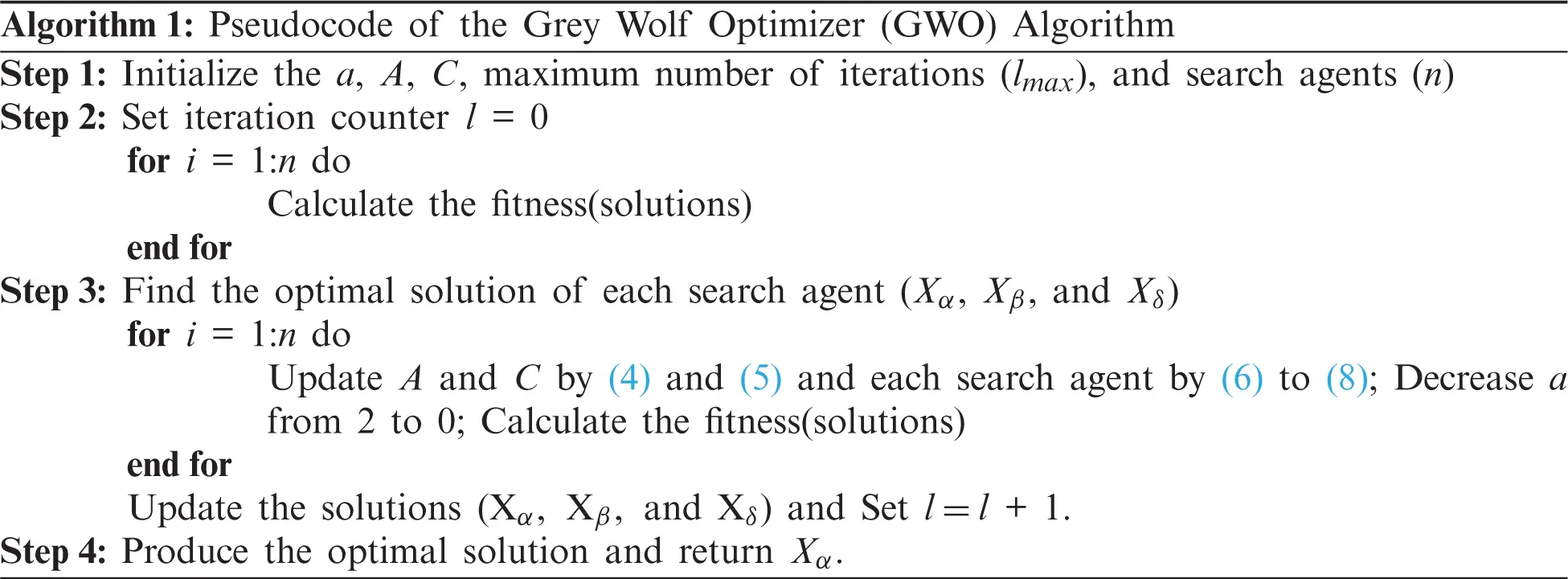Two mechanisms are integrated to formulate the MOGWO algorithm as similar to multi-objective Particle Swarm Optimization (MOPSO). The first component of MOGWO is an archive, and it is accountable for saving the non-dominated best solution obtained until now, and leader selection strategy is the second component that helps to choose the solutions of α, β, and δ as the leaders of the hunting hierarchy. An archive is a storing unit that either stores or retrieves the non-dominated Pareto optimal solution. An archive controller is an archive unit that manages the archive if the solution enters into the archive or if the archive is occupied completely. It is noted that a large number of members are required for an archive. The non-dominated Pareto solution pairs are compared with the members of the archive during the iterations. So, there are three possible cases and are given as follows.

•   The newly obtained solution controls one or more archive solutions. Therefore, the dominated archive solution must be eliminated, and the newer solution enters/adds to the archive

•   If the archive is filled, the grid method is used to reorganize the section/segment of the target space and then to discover the highest crowded section/segment to delete one of its solutions. To increase the diversity or variety of the optimal Pareto front, the newer solution must be added/enter into the less crowded segment/section

•   If both archive members and the new solution are not dominated each other, the newer solution must be entering into the archive.

Suppose the number of solutions in the hypercube is increased, the probability of deleting the solution is also increased. If the archive is full, the highest crowded sections/segments are first chosen, and one of the solutions is eliminated randomly to give space for the newly updated solution. If the solution is introduced outside the hypercubes, all sections/portions are expanded to protect the new results. Thus, the other solutions of the segments can also be modified. The comprehensive details are available in . The Pseudocode of the MOGWO algorithm is given in Algorithm 2. The latter component is the leader selection mechanism. In the basic version of GWO, the solutions provided by the α, β, and δ wolves are considered as the three best solutions attained so far. The α, β, and δ wolves direct the other search agents in promising search space to find the optimal solutions. Nonetheless, solutions cannot easily be contrasted in a multi-objective search space due to the Pareto optimal principles, and the leader selection mechanism is employed to handle this problem. As discussed, the best solutions are stored in an archive that has been attained until now. The leader selection mechanism selects the least crowded search segments and suggests one of its non-dominated wolves, either α, or β, or δ. The selection is performed with the subsequent probability for each hypercube by a roulette wheel process.

Pi=CNi (9)

where N denotes the number of Pareto optimal solutions obtained in the ith section and C represents a constant number, which is higher than one. The MOGWO’s computational complexity is O(AB2), where B is the number of objectives, and A is the number of populations. The complexity of the MOGWO is similar to other famous algorithms, such as MOPSO, PAES, SPEA2, and NSGA-II. Moreover, the complexity is better than SPEA and NSGA. In multi-objective BLDC motor design problems, the MOGWO generates a set of non-dominated solutions with the best trade-off between the mass and efficiency situated on a Pareto front graph. In BLDC motor design, five design variables must be optimized to achieve the best trade-off between total motor mass and efficiency. In this paper, to find the best value of all design parameters by satisfying the design constraints, a multi-objective and mono-objective GWO algorithm is proposed, and its performance is verified by applying the algorithm to the analytical model of the BLDC motor.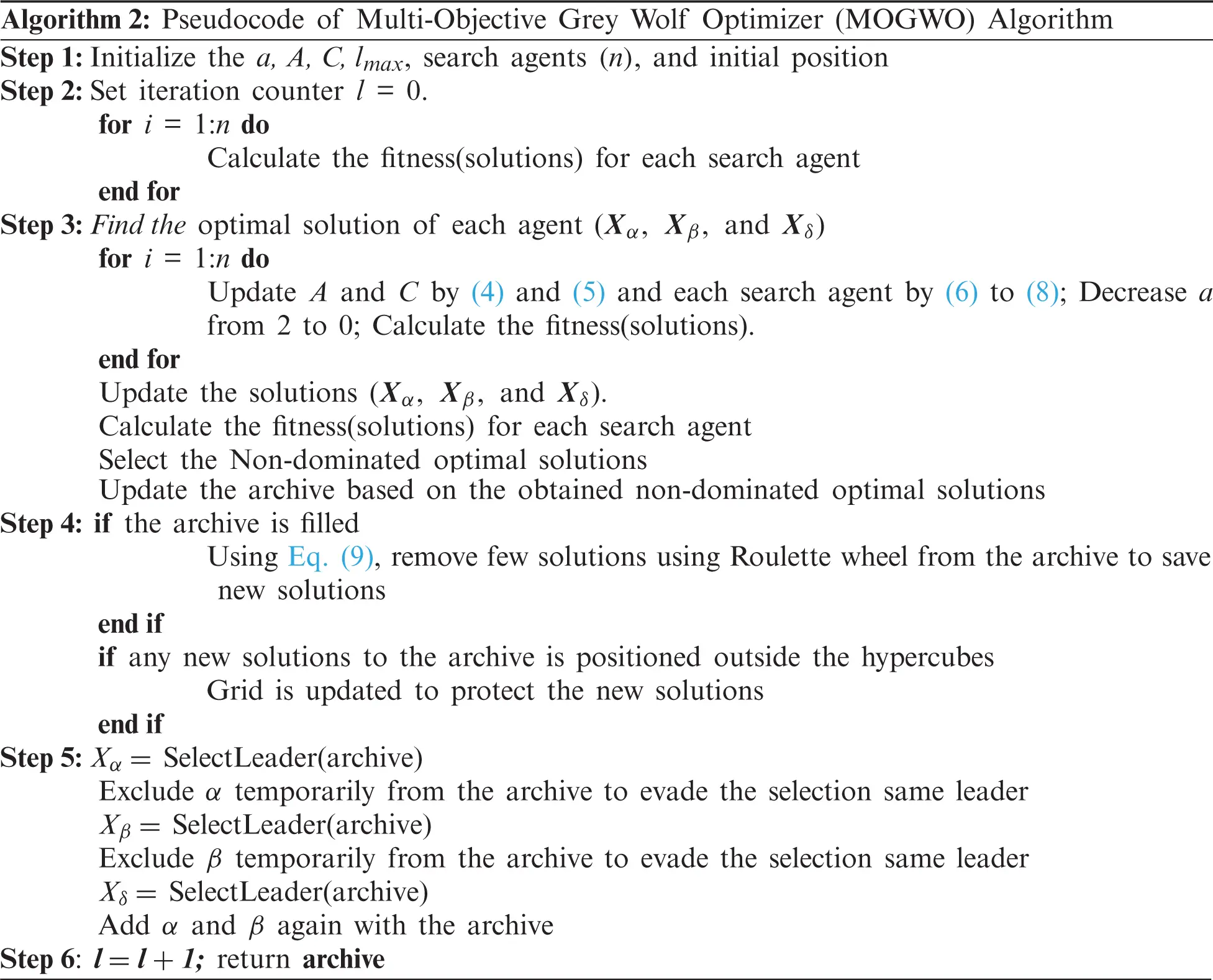4  Simulation Results and Discussions

The MOGWO algorithm optimizes the BLDC motor design variables, and the results obtained by the MOGWO are discussed in this section. The algorithm starts with the initialization of the population size, archive size, maximum number of iterations, and the boundary condition of BLDC motor variables. After that, the initial position, and objective function values are obtained that can proceed through the MOGWO algorithm to find the best optimal tradeoff points between two objective functions in terms of mass and efficiency. The performance of the MOGWO algorithm is firstly verified on four unconstrained multi-objective problems and then applied to the electromagnetic optimization problem. The simulation is carried out using MATLAB software installed on a PC with 4 GB RAM and 3.20 GHz clock frequency. Each unconstraint test benchmark function is solved with 30 independent runs. The control parameters of the proposed MOGWO algorithm are initialized as follows: the population size is equal to 100, the maximum number of iterations is equal to 300, and an archive size is equal to 100. The performance metrics, such as Generational Distance (GD), Inversion Generational Distance (IGD), Hypervolume-Matrix (HV-Matrix), Scattered-Matrix (S-Matrix), and Coverage of all multi-objective algorithms are discussed in this section .

4.1 MOGWO Results for Test Benchmark Problems

Before solving real-world problems, first of all, the MOGWO is applied to the unconstraint test benchmark function suggested in  to verify the performance. Four unconstraint test benchmark functions, ZDT1, ZDT3, ZDT4, and ZDT6, are considered, and a comprehensive simulation is carried out using all selected metaheuristic algorithms. The control parameters of any algorithm play a vital role in the solution of the optimization problem. Therefore, the number of populations is selected after performing a comparative study by considering different population sizes and keeping all other factors constant. After careful study, for unconstrained test benchmark functions, the population size, maximum number of iterations, and archive size are selected as 100, 300, and 500, respectively. The other parameter settings of all selected algorithms are listed in Tab. 1.The performance metrics, such as GD, S-Matrix, HV-Matrix, Coverage, and IGD for convergence measurement, are used to assess the performance of the MOGWO algorithm. Tab. 2 lists all the performance metrics of algorithms, such as NSGA-II, PAES, MOGWO, MOFA, MOBA, MOFPA, MOSFLA, MOPSO, MOALO, MOGOA, and MOSSA. Tab. 2 shows that MOGWO can exhibit the optimal results on all performance metrics. GD, S-matrix, HV-Matrix, IGD, and Coverage show the convergence and solution accuracy of all algorithms. So, it is concluded that the suggested MOGWO can offer the best convergence on all the benchmark functions. Fig. 1 shows the results (archive solutions) of all four test benchmark problems. It can be seen that the MOGWO algorithm can able to approximate the Pareto front. It is also seen that the suggested MOGWO can able to exhibit satisfactory performance by comparing the Pareto front estimates. Therefore, it is concluded that the MOGWO algorithm is more appropriate for BLDC motor parameter design.

4.2 Mono-Objective BLDC Wheel Motor Design Problem

In this context, the BLDC wheel motor is optimized by considering 78 non-linear equations, including electric, magnetic as well as thermal characteristics. Firstly, the MOGWO algorithm is tested for the mono-objective problem, i.e., efficiency maximization. The maximum number of iterations is selected after several trials run and selected as 500. The objective function is evaluated using MATLAB software for various search agents to find the optimal search agent. The results obtained by the MOGWO algorithm for various search agents are demonstrated in Fig. 2, and five optimized parameters are listed in Tab. 3. From Tab. 3, it is decided to select the search agents as 100.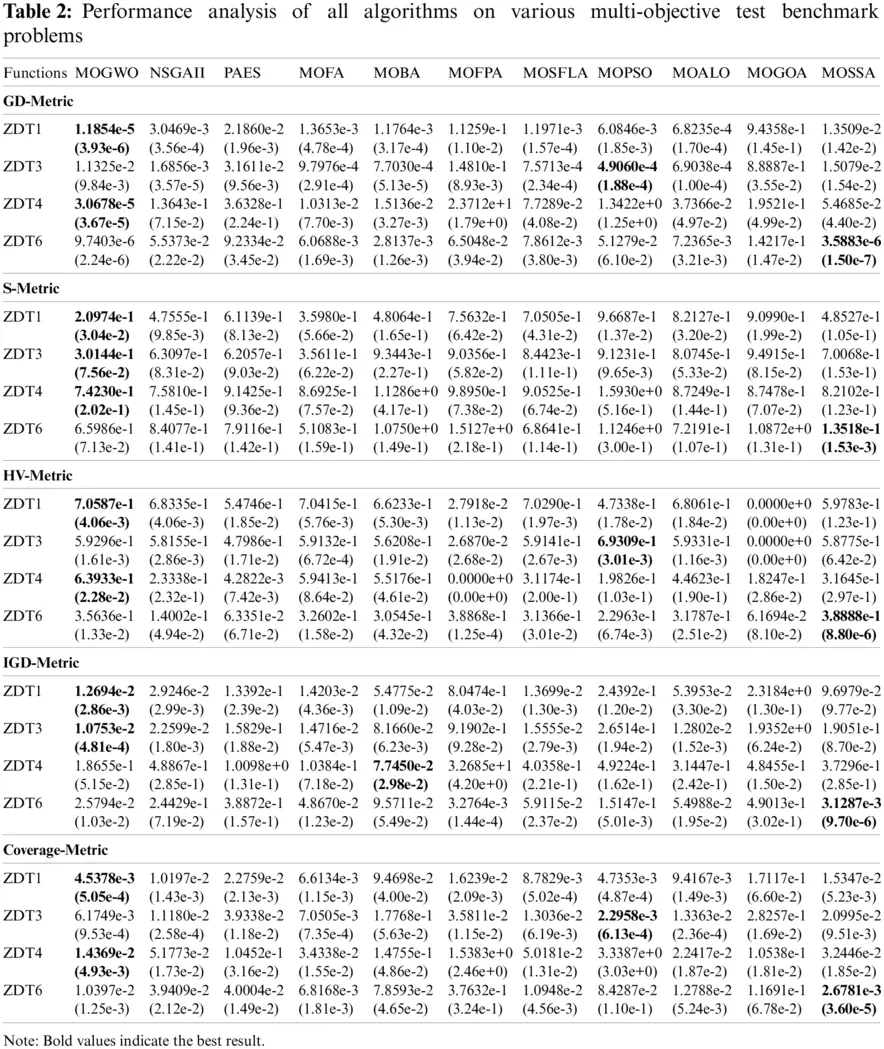Figure 1: Best Pareto optimal front obtained by MOGWO algorithm; (a) ZDT1, (b) ZDT3, (c) ZDT4 and (d) ZDT6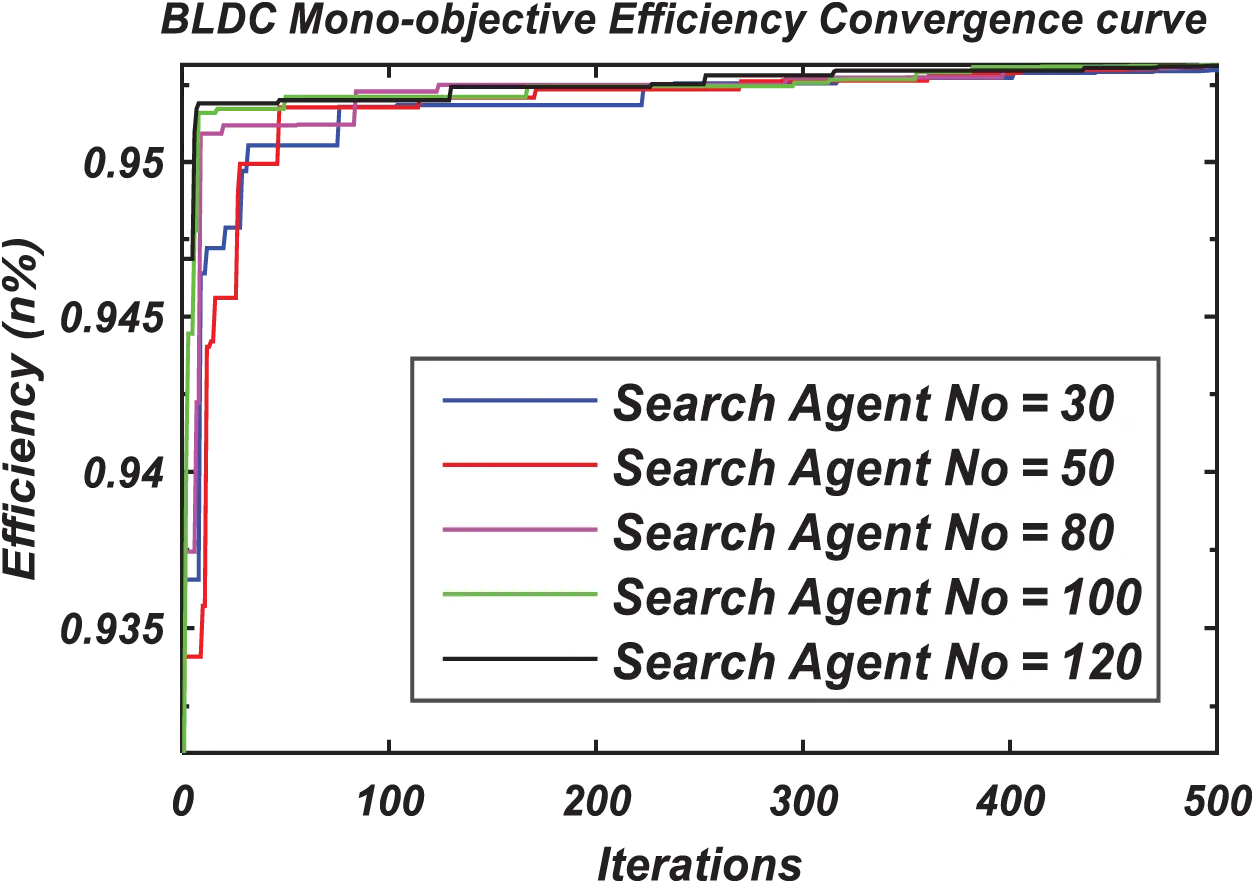Figure 2: Convergence curve for various populations of mono-objective BLDC wheel motor design problem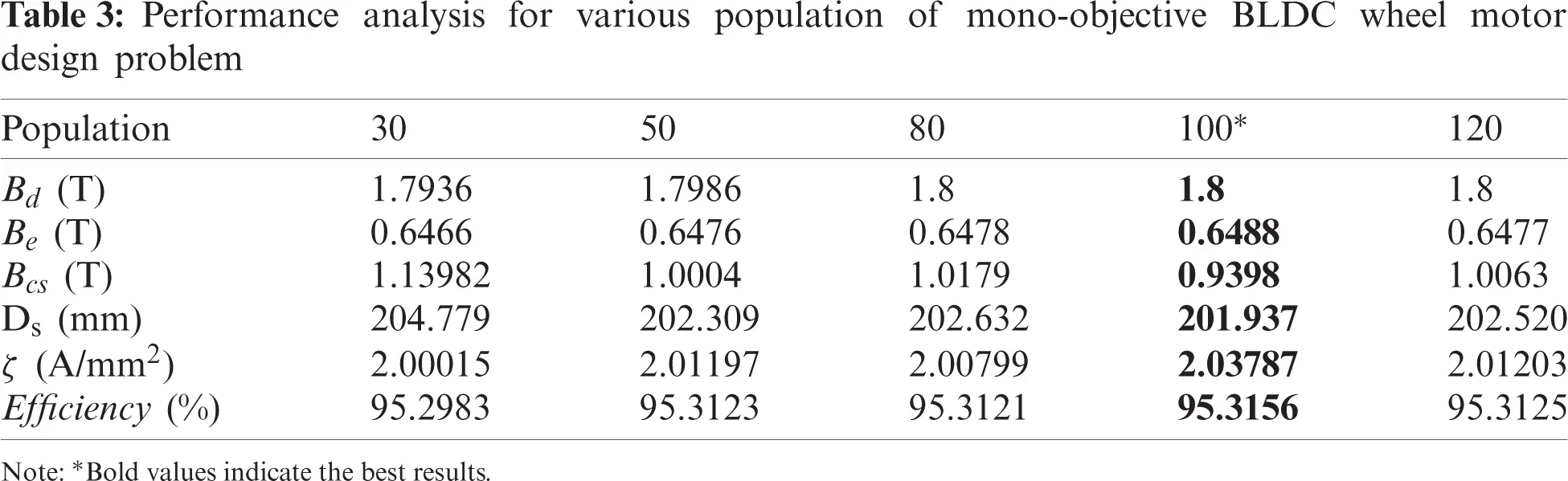After selecting the optimal search agents and the maximum number of iterations, the mono-objective problem is evaluated by various state-of-the-algorithms, such as PSO, GA, Sequential Quadratic Programming (SQP), hybrid SQP-GA, Ant Colony Optimization (ACO), Bat Algorithm (BA), and GWO. The control parameters of all algorithms are selected as similar to the GWO algorithm. Tab. 4 represents the numerical results for mono-objective BLDC wheel motor problems, and a comparison is made among all selected algorithms. The results produced by the GWO are very much close to the best results after 30 independent runs. The results in terms of mean and standard deviation (STD) of mono-objective BLDC motor with 30 independent runs of GWO are presented in Tab. 5. The statistical values of mean and STD for motor efficiency are observed to be very small from Tab. 5.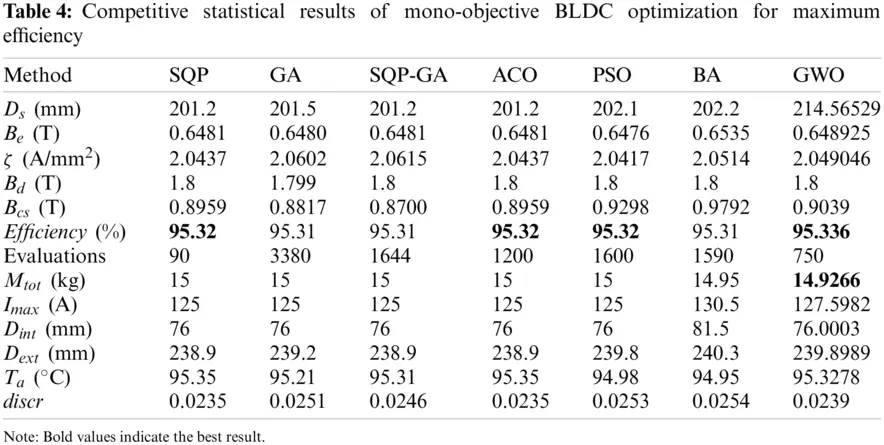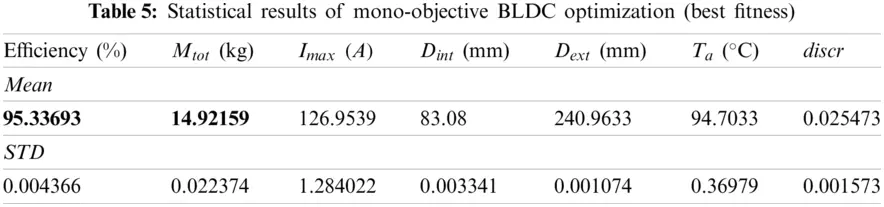4.3 Multi-Objective BLDC Wheel Motor Design Problem

As similar to the mono-objective problem, the multi-objective optimization problem also runs 30 times with an archive size of 100, the maximum number of iterations of 6000, and the population size of 100. The best-compromised results are listed in Tab. 6, in which the best feasible solutions are presented.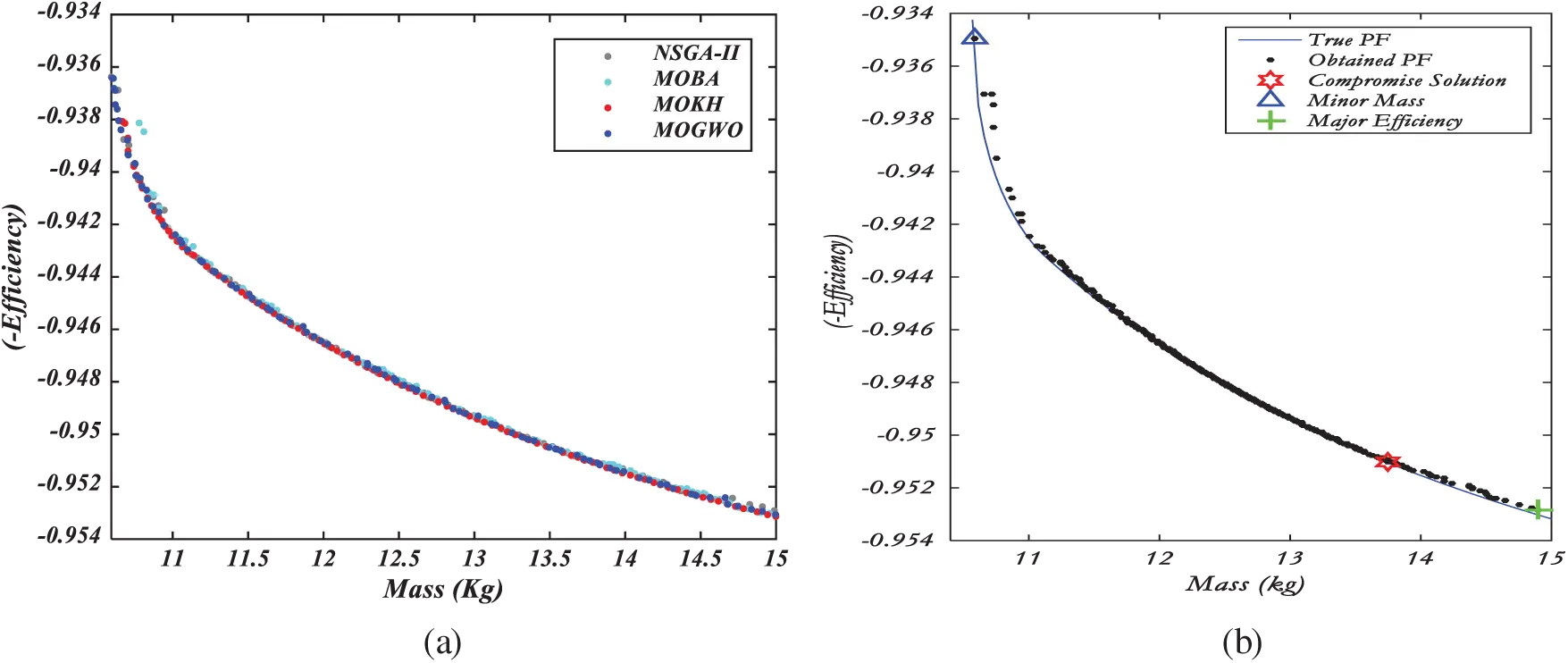Figure 3: Best Pareto optimal front; (a) Various selected algorithms, (b) MOGWO algorithm with the best compromise solution

The best Pareto front of various selected algorithms and the best compromise solution obtained by the MOGWO algorithm is illustrated in Fig. 3. Other state-of-the-art algorithms, such as MOBA, NSGA-II, and MOKH, are also applied to solve the same problem. The statistical results and performance analysis of the multi-objective BLDC problem are shown in Tab. 7. In view of correlating the previous discussions, it is exciting to compare MOGWO with the MOKH, MOBA, and NSGA-II. For this reason, one of the results is analyzed, i.e., the top possible harmonic solution given by the one nearest to the harmonic mean of all Pareto front solutions. The harmonic mean can be determined for mass or efficiency.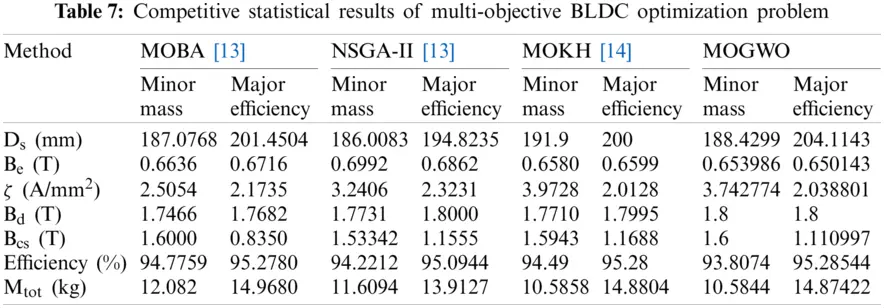After 30 individual runs, Tab. 7 shows the feasible harmonic solution for all selected multi-objective algorithms. The performance is analyzed with respect to the maximization of efficiency and the minimization of mass. With respect to the mass of the motor, the methods, such as MOGWO and NSGA-II, perform better than the other two methods. With respect to both motor mass and motor efficiency, the MOGWO algorithm is very much better with less mass and high efficiency than all the methods, as listed in Tab. 7. The performance metrics, such as GD, S-Matrix, HV-Matrix, IGD, and Coverage for the algorithms, such as NSGA-II, MOBA, and MOGWO, are listed in Tab. 8. From Tab. 8, it is noted and concluded that the suggested MOGWO displays better performance than the other selected algorithms.4.4 Statistical Validation

Wilcoxon signed-rank test  is employed on ZDT unconstrained benchmarks to compare the performance of all selected algorithms at a significant level of 0.05, and the results are shown in Tab. 9. This test is a nonparametric test, and it can be used to find the statistical difference between the two different techniques.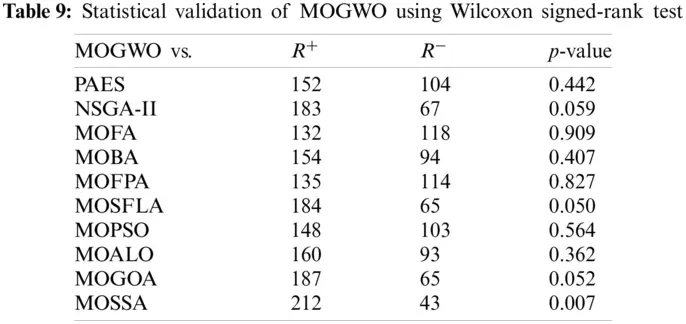The mean values of the metrics over 30 runs on all test functions are utilized as the sample values. Thus, according to the analysis mentioned above, it is concluded that MOGWO can show a competitive and robust performance when compared with PAES, NSGA-II, MOFA, MOBA, MOFPA, MOSFLA, MOPSO, MOALO, MOGOA, and MOSSA.

5  Conclusion

The MOGWO is a new optimization technique that appears to be a robust tool for engineering optimization problems. Furthermore, with respect to the BLDC wheel motor design optimization problem, MOGWO can demonstrate to be competitive compared to other state-of-the-art optimization methods. The numerical results obtained by the MOGWO algorithm for BLDC wheel motor design are compared with the other swarm and evolutionary-based computational techniques. The performance measurement in multi-objective optimization in terms of convergence, coverage, and success matrices for BLDC motor design with MOGWO has been acquired, and statistical results demonstrated the effectiveness of MOGWO in the field of electromagnetic optimization application. The outcomes display a good trend for the MOGWO in both mono-objective and multi-objective optimization problems. The motor efficiency is nearer to 95.285% while solving the BLDC wheel motor design problem using the MOGWO algorithm. The results obtained using MOGWO is depending on the control parameters tuning. The MOGWO can also be applied to other optimization problems, such as optimal power flow, economic load dispatch, transformer design, etc. In future research, the same concept can be extended for other specification sheets, such as ten parameter and eleven parameter problems.

Acknowledgement: The authors would like to thank GMR Institute of Technology, Rajam, Andhra Pradesh, India, for providing a laboratory facility to conduct experiments.

Funding Statement: The authors received no specific funding for this study.

Conflicts of Interest: The authors declare that they have no conflicts of interest to report regarding the present study.

## References

1. C. Jui-Hung and L. Shuo-Fang, “Simulation and design optimization of permanent magnet brushless DC motors,” Journal of Interdisciplinary Mathematics, vol. 20, no. 3, pp. 777–788, 2017.
2. M. Premkumar, J. S. V. Sivakumar, R. Vijaya Krishna and R. Sowmya, “Design, analysis and fabrication of solar PV powered BLDC hub motor driven electric car,” International Journal of Mechanical and Production Engineering Research and Development, vol. 8, no. 1, pp. 1255–1270, 2018.
3. K. S. Rama Rao and A. H. B. Othman, “Design optimization of a BLDC motor by Genetic Algorithm and Simulated Annealing,” in Proc. of Int. Conf. on Intelligent and Advanced Systems, Kuala Lumpur, pp. 854–858, 2007.
4. A. Shabanian, A. Amini, P. Tousiwas, M. Pourmandi, A. Khormali et al., “Optimization of brushless direct current motor design using an intelligent technique,” ISA Transactions, vol. 57, pp. 311–321, 2015.
5. E. Fitan, F. Messine and B. Nogarede, “The electromagnetic actuator design problem: A general and rational approach,” IEEE Transactions on Magnetics, vol. 40, no. 3, pp. 1579–1590, 2004.
6. A. Sarikhani and O. A. Mohammed, “Multiobjective design optimization of coupled PM synchronous motor-drive using physics-based modeling approach,” IEEE Transactions on Magnetics, vol. 47, no. 5, pp. 1266–1269, 2011.
7. T. Back, D. Fogel and Z. Michalewicz, “Evolutionary computation models” in Handbook of Evolutionary Computation, vol. F1. New York, USA: Oxford University Press, pp. F1.2:1–F1.2:50, 199
8. S. Das, S. Maity, B. Y. Qu and P. N. Suganthan, “Real-parameter evolutionary multimodal optimization—A survey of the state-of-the-art,” Swarm and Evolutionary Computation, vol. 1, no. 2, pp. 71–88, 2011.
9. J. Kennedy, R. C. Eberhart and Y. Shi, “Humans-actual, imagined, and implied,” in Swarm Intelligence. San Francisco, USA: Morgan Kaufmann Publisher, pp. 187–258, 2001.
10. A. P. Engelbrecht, “Evolutionary computation paradigms,” in Fundamentals of Computational Swarm Intelligence. Hoboken, USA: John Wiley & Sons, pp. 450–485, 2006.
11. X. S. Yang, Classic optimization methods-II. In: Engineering Optimization: An Introduction with Metaheuristic Applications. United Kingdom: John Wiley & Sons, pp. 45–95, 2010.
12. S. Yang, J. M. Machado, N. Guangzheng, S. L. Ho and P. Zhou, “A self-learning simulated annealing algorithm for global optimizations of electromagnetic devices,” IEEE Transactions on Magnetics, vol. 36, no. 4, pp. 1004–1008, 2000.
13. T. C. Bora, L. D. S. Coelho and L. Lebensztajn, “Bat-inspired optimization approach for the brushless dc wheel motor problem,” IEEE Transactions on Magnetics, vol. 48, no. 2, pp. 947–950, 2012.
14. H. V. H. Ayala, E. H. V. Segundo, V. C. Mariani and L. D. S. Coelho, “Multiobjective krill herd algorithm for electromagnetic optimization,” IEEE Transactions on Magnetics, vol. 52, no. 3, pp. 1–4, 2016.
15. L. D. Santos Coelho, L. Z. Barbosa and L. Lebensztajn, “Multiobjective particle swarm approach for the design of a brushless dc wheel motor,” IEEE Transactions on Magnetics, vol. 46, no. 8, pp. 2994–2997, 2010.
16. A. Fanni, A. Manunza, M. Marchesi and F. Pilo, “Tabu search metaheuristics for electromagnetic problems optimization continuous domains,” IEEE Transactions on Magnetics, vol. 35, no. 3, pp. 1694–1697, 1999.
17. Y. D. Chun, S. Wakao, T. H. Kim, K. B. Jang and J. Lee, “Multiobjective design optimization of brushless permanent magnet motor using 3D equivalent magnetic circuit network method,” IEEE Transactions on Applied Superconductivity, vol. 14, no. 2, pp. 1910–1913, 2004.
18. P. S. Shin, H. D. Kim, G. B. Chung, H. S. Yoon, G. S. Park et al., “Shape optimization of large-scale BLDC motor using an adaptive RSM utilizing design sensitivity analysis,” IEEE Transactions on Magnetics, vol. 43, no. 4, pp. 1653–1656, 2007.
19. K. J. Han, H. S. Cho, D. H. Cho and H. K. Yung, “Optimal core shape design for cogging torque reduction of brushless DC motor using genetic algorithm,” IEEE Transactions on Magnetics, vol. 36, no. 4, pp. 1927–1931, 2006.
20. S. Mirjalili, S. M. Mirjalili and A. Lewis, “Grey wolf optimizer,” Advances in Engineering Software, vol. 69, pp. 41–61, 2014.
21. S. Mirjalili, S. Saremi, S. M. Mirjalili and L. D. S. Coelho, “Multi-objective grey wolf optimizer: A novel algorithm for multi-criterion optimization,” Expert Systems with Applications, vol. 47, pp. 106–119, 2016.
22. J. Le Besnerais, V. Lanfranchi, M. Hecquet and P. Brochet, “Multiobjective optimization of induction machines including mixed variables and noise minimization,” IEEE Transactions on Magnetics, vol. 44, no. 6, pp. 1102–1105, 2008.
23. S. S. Arshi, A. Zolfaghari and S. Mirvakili, “A multi-objective shuffled frog leaping algorithm for in-core fuel management optimization,” Computer Physics Communications, vol. 185, pp. 2622–2628, 2014.
24. S. Mirjalili, P. Jangir and S. Saremi, “Multi-objective ant lion optimizer: A multi-objective optimization algorithm for solving engineering problems,” Applied Intelligence, vol. 46, pp. 79–95, 2016.
25. S. Z. Mirjalili, S. Mirjalili, S. Saremi, H. Fatis and H. Aljarah, “Grasshopper optimization algorithm for multi-objective optimization problems,” Applied Intelligence, vol. 48, pp. 805–820, 2017.
26. S. Mirjalili, A. H. Gandomi, S. Z. Mirjalili, S. Saremi, H. Faris et al., “Salp swarm algorithm: A bio-inspired optimizer for engineering design problems,” Advances in Engineering Software, vol. 114, pp. 163–191, 2017.
27. G. G. Tejani, S. Kumar and A. H. Gandomi, “Multi-objective heat transfer search algorithm for truss optimization,” Engineering with Computers, vol. 37, pp. 641–662, 2021.
28. G. G. Tejani, N. Pholdee, S. Bureerat, D. Prayogo and A. H. Gandomi, “Structural optimization using multi-objective modified adaptive symbiotic organisms search,” Expert Systems with Applications, vol. 125, pp. 425–441, 2019.
29. S. Kumar, G. G. Tejani, N. Pholdee, S. Bureerat and P. Mehta, “Hybrid heat transfer search and passing vehicle search optimizer for multi-objective structural optimization,” Knowledge-Based Systems, vol. 212, pp. 106556, 2021.
30. S. Kumar, G. G. Tejani, N. Pholdee and S. Bureerat, “Multi-objective modified heat transfer search for truss optimization,” Engineering with Computers, pp. 1–16, 2020. https://doi.org/10.1007/s00366-020-01010-1.
31. S. Kumar, G. G. Tejani, N. Pholdee and S. Bureerat, “Multi-objective passing vehicle search algorithm for structure optimization,” Expert Systems with Applications, vol. 169, pp. 114511, 2021.
32. M. Premkumar, P. Jangir, R. Sowmya, H. H. Alhelou, A. A. Heidari et al., “MOSMA: Multi-objective slime mould algorithm based on elitist non-dominated sorting,” IEEE Access, vol. 9, pp. 3229–3248, 2021.
33. M. Premkumar, P. Jangir and R. Sowmya, “MOGBO: A new multiobjective gradient-based optimizer for real-world structural optimization problems,” Knowledge-Based Systems, vol. 218, pp. 106856, 2021.
34. M. Premkumar, R. Sowmya, P. Jangir, K. Sooppy Nisar and M. Aldhaifallah, “A new metaheuristic optimization algorithms for brushless direct current wheel motor design problem,” Computers, Materials & Continua, vol. 67, no. 2, pp. 2227–2242, 2021.
35. S. Brisset and P. Brochet, “Analytical model for the optimal design of a brushless DC wheel motor,” COMPEL The International Journal for Computation and Mathematics in Electrical and Electronic Engineering, vol. 24, no. 3, pp. 829–848, 2005.
36. S. Brisset, “Lille laboratory of electrical engineering and power electronics (L2EP),” Analytical Model, Benchmark Functions, 2005. [Online] Available: http://l2ep.univ-lille1.fr/come/benchmark-wheel-motor/Math.htm [Accessed on June 2020].
37. S. Mirjalili and A. Lewis, “Novel performance metrics for robust multi-objective optimization algorithms,” Swarm and Evolutionary Computation, vol. 21, pp. 1–23, 2015.
38. E. Zitzler, K. Deb and L. Thiele, “Comparison of multiobjective evolutionary algorithms: Empirical results,” Evolutionary Computation, vol. 8, no. 2, pp. 173–195, 2000.
39. J. Derrac, S. García, D. Molina and F. Herrera, “A practical tutorial on the use of nonparametric statistical tests as a methodology for comparing evolutionary and swarm intelligence algorithms,” Swarm and Evolutionary Computation, vol. 1, no. 7, pp. 3–18, 2011.This work is licensed under a Creative Commons Attribution 4.0 International License, which permits unrestricted use, distribution, and reproduction in any medium, provided the original work is properly cited.